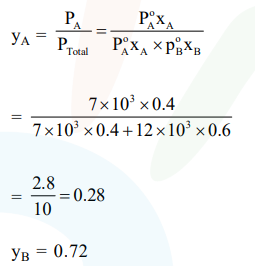# Liquids A and B form an ideal solution in the entire`
Question:

Liquids A and B form an ideal solution in the entire composition range. At $350 \mathrm{~K}$, the vapor pressures of pure A and pure $\mathrm{B}$ are $7 \times 10^{3} \mathrm{~Pa}$ and $12 \times 10^{3} \mathrm{~Pa}$, respectively. The composition of the vapor in equilibrium with a solution containing 40 mole percent of A at this temperature is:

1. $\mathrm{x}_{\mathrm{A}}=0.37 ; \mathrm{x}_{\mathrm{B}}=0.63$

2. $\mathrm{x}_{\mathrm{A}}=0.28 ; \mathrm{x}_{\mathrm{B}}=0.72$

3. $\mathrm{x}_{\mathrm{A}}=0.76 ; \mathrm{x}_{\mathrm{B}}=0.24$

4. $\mathrm{x}_{\mathrm{A}}=0.4 ; \mathrm{x}_{\mathrm{B}}=0.6$

Correct Option: , 2

Solution: